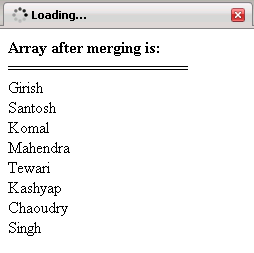Tutorials

# JavaScript array merge

In this Tutorial we want to describe you a code that help you in understanding how to merge two different array into one array using Java Script.

In this Tutorial we want to describe you a code that help you in understanding how to merge two different array into one array using Java Script.

# JavaScript array merge

In this Tutorial we want to describe you a code that help you in understanding how to merge two different array into one array using Java Script. We declare a array variable that is used to store array object. An array component  is accessed by an array expression that consists of an expression whose value is an array reference indicated by subscripts indexing expression enclosed by `[]`. An array with size length of x can be indexed by the integers from `0` to x`-1`.The document. write prints the elements in an array. The for loop execute the script till the array variable is less than array length.The document. write print the list of element in an array. In the same way we declare another array variable array1 that is used to used to store an array object and each array reference hold the list of element in array1 variable indicated by the indexes. The document.write print the list of element in array.

On clicking a button the function1( )is invoked that include

1)array.concat(array1) - concatenated the two array and store in a variable c

Finally the document. write print the concatenated array by merging two array.

Arraymerge.html

 ```     Array Declaration            function function1(){   var c=array.concat(array1);   document.write(""+"Array after merging is:    "+""+"
");   document.write("===================="+"
");   for(var i=0;i")   }    }    var array=new Array();   array="Girish";   array="Santosh";   array="Komal";   array="Mahendra";   document.write(""+"Element in the array are    "+""+ "");   document.write("================="+ "");   for(var i=0;i")   }      var array1=new Array();   array1="Tewari";   array1="Kashyap";   array1="Chaoudry";   array1="Singh";   document.write(""+"Element in the array are    "+""+ "");   document.write("================="+ "");   for(var j=0;j")   }   document.write("");          Merge Arrays    ```

Output of the program